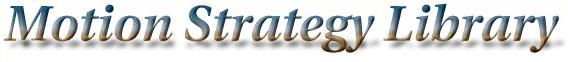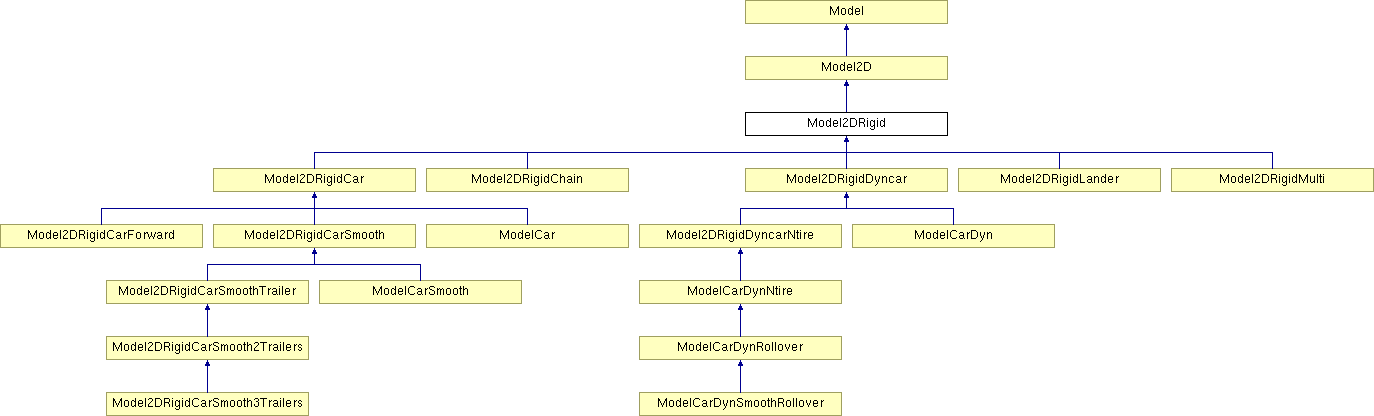Main Page   Namespace List   Class Hierarchy   Compound List   File List   Compound Members   File Members

# Model2DRigid Class Reference

A holonomic rigid robot in a 2D world. More...

#include <model2d.h>

Inheritance diagram for Model2DRigid:List of all members.

## Public Methods

Model2DRigid (string path)
virtual ~Model2DRigid ()
virtual MSLVector Integrate (const MSLVector &x, const MSLVector &u, const double &h)
Perform integration from state x, using input u, over time step h. More...

virtual MSLVector StateTransitionEquation (const MSLVector &x, const MSLVector &u)
The state transition equation, or equations of motion, xdot=f(x,u). More...

MSLVector LinearInterpolate (const MSLVector &x1, const MSLVector &x2, const double &a)
Linearly interpolate two state while respecting topology. More...

virtual MSLVector StateDifference (const MSLVector &x1, const MSLVector &x2)
Compute a MSLVector based on x2-x1. In R^n, the states are simply subtracted to make the MSLVector. This method exists to make things work correctly for other state-space topologies. More...

virtual double Metric (const MSLVector &x1, const MSLVector &x2)
A distance metric, which is Euclidean in the base class. More...

virtual MSLVector StateToConfiguration (const MSLVector &x)
A method that converts a Model state in to a Geom configuration. More...

## Detailed Description

A holonomic rigid robot in a 2D world.

## Constructor & Destructor Documentation

 Model2DRigid::Model2DRigid ( string path )

 virtual Model2DRigid::~Model2DRigid ( )  [inline, virtual]

## Member Function Documentation

 MSLVector Model2DRigid::Integrate ( const MSLVector & x, const MSLVector & u, const double & h )  [virtual]
 Perform integration from state x, using input u, over time step h. Implements Model. Reimplemented in Model2DRigidDyncar.

 MSLVector Model2DRigid::LinearInterpolate ( const MSLVector & x1, const MSLVector & x2, const double & a )  [virtual]
 Linearly interpolate two state while respecting topology. If a=0, then x1 is returned; if a=1, then x2 is returned. All intermediate values of $a \in [0,1]$ yield intermediate states. This method is defined by Model. Reimplemented from Model. Reimplemented in Model2DRigidDyncar.

 double Model2DRigid::Metric ( const MSLVector & x1, const MSLVector & x2 )  [virtual]
 A distance metric, which is Euclidean in the base class. Reimplemented from Model. Reimplemented in Model2DRigidCarSmooth.

 MSLVector Model2DRigid::StateDifference ( const MSLVector & x1, const MSLVector & x2 )  [virtual]
 Compute a MSLVector based on x2-x1. In R^n, the states are simply subtracted to make the MSLVector. This method exists to make things work correctly for other state-space topologies. Reimplemented from Model. Reimplemented in Model2DRigidDyncar.

 MSLVector Model2DRigid::StateToConfiguration ( const MSLVector & x )  [virtual]
 A method that converts a Model state in to a Geom configuration. Reimplemented from Model2D. Reimplemented in Model2DRigidCarSmooth.

 MSLVector Model2DRigid::StateTransitionEquation ( const MSLVector & x, const MSLVector & u )  [virtual]
 The state transition equation, or equations of motion, xdot=f(x,u). Implements Model. Reimplemented in Model2DRigidCar.

The documentation for this class was generated from the following files: Motion Strategy Library

Web page maintained by Steve LaValle